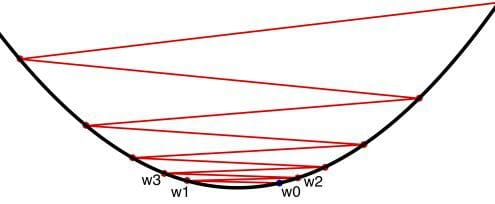Topics: AI | Data Science | Data Visualization | Deep Learning | Machine Learning | NLP | Python | R | Statistics

KDnuggets Home » News » 2016 » Oct » Tutorials, Overviews » Deep Learning Key Terms, Explained ( 16:n37 )

#Deep Learning Key Terms, Explained

http likes 1152

Gain a beginner's perspective on artificial neural networks and deep learning with this set of 14 straight-to-the-point related key concept definitions, including Biological Neuron, Multilayer Perceptron (MLP), Feedforward Neural Network, and Recurrent Neural Network.

6. Feedforward Neural Network

Feedforward neural networks are the simplest form of neural network architecture, in which connections are non-cyclical. The original artificial neural network, information in a feedforward network advances in a single direction from the input nodes, though any hidden layers, to the output nodes; no cycles are present. Feedforward networks differ from later, recurrent network architectures (see below), in which connections form a directed cycle.

7. Recurrent Neural Network

In contrast to the above feedforward neural networks, the connections of recurrent neural networks form a directed cycle. This bidirectional flow allows for internal temporal state representation, which, in turn, allows sequence processing, and, of note, provides the necessary capabilities for recognizing speech and handwriting.

8. Activation Function

In neural networks, the activation function produces the output decision boundaries by combining the network's weighted inputs. Activation functions range from identity (linear) to sigmoid (logistic, or soft step) to hyperbolic (tangent) and beyond. In order to employ backpropagation (see below), the network must utilize activation functions which are differentiable.9. Backpropagation

The best concise, elementary definition of backpropagation I have ever come across was by data scientist Mikio L. Braun, giving the following answer on Quora, which I reproduce verbatim so as not soil its simple perfection:

Back prop is just gradient descent on individual errors. You compare the predictions of the neural network with the desired output and then compute the gradient of the errors with respect to the weights of the neural network. This gives you a direction in the parameter weight space in which the error would become smaller.

I'll leave it at that.

10. Cost Function

When training a neural network, the correctness of the network's output must be assessed. As we know the expected correct output of training data, the output of training can be compared. The cost function measures the difference between actual and training outputs. A cost of zero between the actual and expected outputs would signify that the network has been training as would be possible; this would clearly be ideal.

So, by what mechanism is the cost function adjusted, with a goal of minimizing it?

Gradient descent is an optimization algorithm used for finding local minima of functions. While it does not guarantee a global minimum, gradient descent is especially useful for functions which are difficult to solve analytically for precise solutions, such as setting derivatives to zero and solving.As alluded to above, in the context of neural networks, stochastic gradient descent is used to make informed adjustments to your network's parameters with the goal of minimizing the cost function, thus bringing your network's actual outputs closer and closer, iteratively, to the expected outputs during the course of training. This iterative minimization employs calculus, namely differentiation. After a training step, the network weights receive updates according the gradient of the cost function and the network's current weights, so that the next training step's results may be a little closer to correct (as measured by a smaller cost function). Backpropagation (backward propagation of errors) is the method used to dole these updates out to the network.

Backpropagation uses the chain rule to compute gradients (by differentiation), in that layers toward the "front" (input) of an n-layer neural network would have their small number updated gradient value multiplied n times before having this settled value used as an update. This means that the the gradient would decrease exponentially, a problem with larger values of n, and front layers would take increasingly more time to train effectively.

13. Convolutional Neural Network

Typically associated with computer vision and image recogntion, Convolutional Neural Networks (CNNs) employ the mathematical concept of convolution to mimic the neural connectivity mesh of the biological visual cortex.

First, convolution, as nicely described by Denny Britz, can be thought of as a sliding window over top a matrix representation of an image (see below). This allows for the loose mimicking of the overlapping tiling of the biological visual field.Credit: Stanford

Implementation of this concept in the architecture of the neural network results in collections of neurons dedicated to processing image sections, at least when employed in computer vision. When utilized in some other domain, such as natural language processing, the same approach can be used, given that input (words, sentences, etc.) could be arranged in matrices and processed in similar fashion.

14. Long Short Term Memory Network (LSTM)Credit: Christopher Olah

A Long Short Term Memory Network (LSTM) is a recurrent neural network which is optimized for learning from and acting upon time-related data which may have undefined or unknown lengths of time between events of relevance. Their particular architecture allows for persistence, giving the ANN a "memory." Recent breakthroughs in handwriting recognition and automatic speech recognition have benefited from LSTM networks.

Related:

Top Stories Past 30 Days
Most SharedGet KDnuggets, a leading newsletter on AI, Data Science, and Machine Learning